## A cube has a drag coefficient of 0.8. What would be the terminal velocity (in m/s) of a jumbo sugar cube 5-cm per side in air. The density o

Question

A cube has a drag coefficient of 0.8. What would be the terminal velocity (in m/s) of a jumbo sugar cube 5-cm per side in air. The density of air is 1.2 kg/m3 and the density of sugar is 1600 kg/m3. Round your answer to the nearest tenth.

in progress 0
1 month 2021-08-13T20:33:45+00:00 2 Answers 0 views 0

The terminal velocity of the sugar would be 40.4 m/s

Explanation:

Given L as the length of the cube = 0.05 m

Area of the sugar =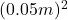= 0.0025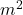The volume of the cube =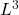=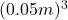= 0.000125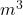Mass of the sugar = Density x volume  =1600 kg/x 0.000125

The terminal velocity of a body can be calculated with the expression below;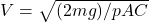Where;

m is the mass of the body = 0.2 kg

g is the acceleration due to gravity = 9.81 m/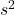p is the density of the fluid (air) = 1.2 kg/A is the area of the body = 0.0025Given L as the length of the cube = 0.05 m

Volume of the cube ==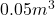= 0.000125Substituting the values in the equation, we have;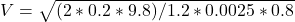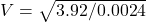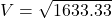V = 40.4 m/s to the nearest tenth

Therefore the terminal velocity of the sugar would be

40.4 m/s

Explanation:

Formula for Terminal Velocity is given as :

• v =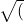2 x mass x acceleration (g) / ( density x area x drag coefficient) )

Mass of sugar =  Density x Volume

Volume of cube = 5cm x 5cm x 5cm = 125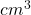= 0.000125Mass of sugar cube = 1600 kg/x 0.000125= 0.2 kg

Area = 5cm x 5 cm = 25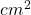= 0.0025V =2 x 0.2 x 9.81 )/ (1600 x 0.0025 x 0.8)

V =3.924/32) =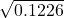V= 0.350 m/s